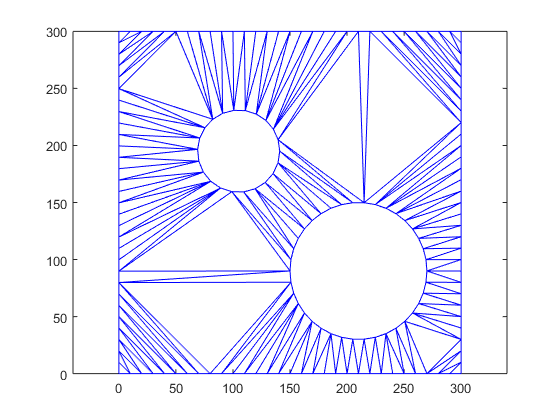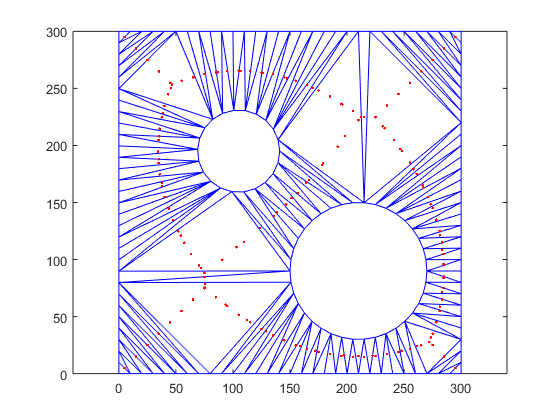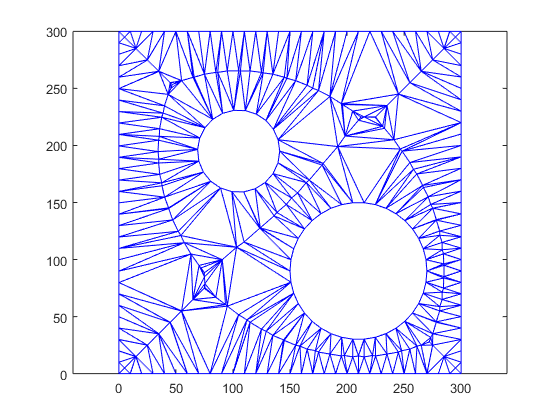## Loren on the Art of MATLABTurn ideas into MATLAB

Note

Loren on the Art of MATLAB has been archived and will not be updated.

# Incremental Delaunay Construction

I'm happy to welcome back Damian Sheehy as guest blogger. Last time Damian wrote about how Natural Neighbor interpolation addresses FAQs in scattered data interpolation. In this blog he will answer a FAQ on adaptively editing a Delaunay triangulation.

### Contents

#### Is delaunayTriangulation More Efficient than delaunay?

A technical support question that occasionally crops up asks about the best and most efficient way to construct a Delaunay triangulation by adding points to an existing triangulation. We call this operation incremental editing as the goal is to add or remove points in an incremental manner as opposed to recreating from scratch; for example calling delaunay multiple times. The documentation for the delaunayTriangulation class provides examples that show the syntax that allows you to edit a Delaunay triangulation by adding or removing points. Let's look at a simple 2-D example to highlight the concept.

In this example we will load trimesh2d.mat which ships with MATLAB. The file contains points with coordinates (x, y) and triangulation edge constraints that define the boundary of a domain. Let's triangulate the data and take a look at that.

load trimesh2d
dt = delaunayTriangulation(x,y,Constraints);
tin = find(dt.isInterior());
triplot(dt(tin,:),dt.Points(:,1),dt.Points(:,2))
axis equalSuppose we want to add the circumcenter points to this triangulation. The circumcenter of a triangle is the center of a circumscribed circle that passes through the vertices of the triangle. The delaunayTriangulation class provides a method to compute them - delaunayTriangulation/circumcenter. Compute them using this method and add them to the plot. Note, some triangles may share the same circumcenter, so I will call the uniquetol function to eliminate the near-duplicates.

cc = dt.circumcenter(tin);
cc = uniquetol(cc, 'ByRows',true);
hold on
plot(cc(:,1),cc(:,2),'.r')
hold offNow, I will add the circumcenter points to the triangulation and plot the result in a new figure.

numcc = size(cc,1);
dt.Points(end+(1:numcc),:) = cc;
tin = find(dt.isInterior());
figure
triplot(dt(tin,:),dt.Points(:,1),dt.Points(:,2))
axis equalSo that's basically an incremental change we made to the triangulation. When prototyping applications like this at the command line we may find adding a few points to a large triangulation can take almost as long as creating the full triangulation of all the points. Why is that, surely the operation should be more efficient?

#### When is Incremental Delaunay Important?

There are some practical applications that rely on this expected level of efficiency. For example, an incremental algorithm for 2-D Mesh Generation using Ruppert's Algorithm. Delaunay-based algorithms for reconstructing surfaces from point clouds may also be incremental; triangulating an initial set of points and adaptively adding more points to recover the surface. In fact the additive points in these algorithms are often circumcenters and that's why I chose them in the example. But the question remains, shouldn't an incremental addition of a few points to a large triangulation be more efficient than a complete triangulation of all points. Absolutely, this behavior is honored in the scenario where algorithms written in MATLAB are designed to run most efficiently. If we write the algorithm in a function in a file, the incremental change will be performed efficiently. If we prototype at the command line the performance we get may underperform the efficiency we get from the function-in-a-file format.

#### Performance Example of Incremental Delaunay Construction

Let's run a little example to test this; the following code creates a 3-D Delaunay triangulation of a half-million points and subsequently adds 40K points in 4 incremental updates. Here's the output when the code runs at the command line:

timeToCreateDelaunay =

   9.7799

  10.1267

When I put the code in a function in a file, execution gives the following:

function DelaunayIncrementalTest()
tic;
dt = delaunayTriangulation(rand(500000,3));
timeToCreateDelaunay = toc

tic;
dt.Points(end+(1:10000),:) = rand(10000,3);
dt.Points(end+(1:10000),:) = rand(10000,3);
dt.Points(end+(1:10000),:) = rand(10000,3);
dt.Points(end+(1:10000),:) = rand(10000,3);
end


DelaunayIncrementalTest()

timeToCreateDelaunay =
9.9275
1.1396


Why the significant performance improvement? MATLAB can execute code more efficiently when it is in the function-in-file format. Loren has a past blog on In-place Operations that highlights the behavior that improves the efficiency here. So to gauge the performance of your MATLAB code it's good to structure your code into functions that reside in files. Then run the performance analyzer and eliminate the bottlenecks.

#### Your Need for Geometric Tools?

Does your work involve triangulations or geometric computing? Does your application area require geometric tools or features that are not well supported in core MATLAB? Close the loop and share your experience here; hearing what users' need is the compass that charts our feature enhancements!

Published with MATLAB® R2015a